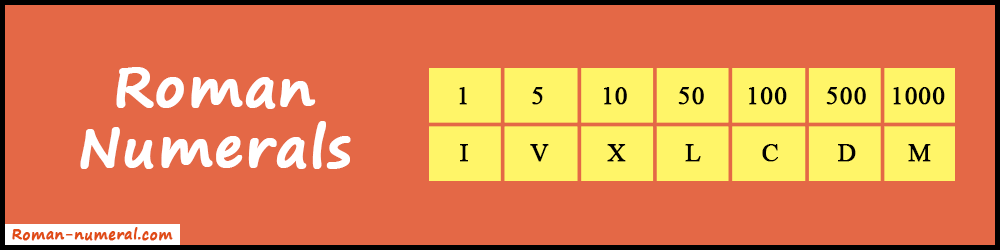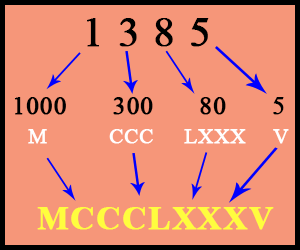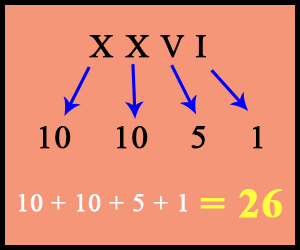# Roman Numerals

Here you will find commonly used and some basic combinations of Roman Numerals with forming rules and big Roman numbers.

## Commonly used Roman Numerals

 1 5 10 50 100 500 1000 I V X L C D M

#### Some Basic Combinations

 1 2 3 4 5 6 7 8 9 I II III IV V VI VII VIII IX
 10 20 30 40 50 60 70 80 90 X XX XXX XL L LX LXX LXXX XC
 100 200 300 400 500 600 700 800 900 C CC CCC CD D DC DCC DCCC CM

#### Forming Rules

When a symbol is placed after a greater (or equal) value then it is added.

• Example: LX = L + X = 50 + 10 = 60

Similarly, when a symbol is placed before a greater value then it is subtracted.

• Example: XC = C - X = 100 - 10 = 90

Note: Do not use the same symbol more than three times in one row. But sometimes IIII is used to show 4 in clocks.

#### Big Numbers

Arabic numbers more than 1000 are defined by placing a bar (─) over the symbol or letter. Also, it multiplies its value by 1000.

 5000 10000 50000 100000 500000 1000000 V X L C D M
##### You may also like:
Roman Numerals Converter Date to Roman Numerals

### What are Roman Numerals?

Roman Numerals are the ancient Roman way of representing numbers. Just like today as we have the modern numeral system, Romans had their own system of representing numbers. It's one of the oldest ways but still, it's widely used. You can easily find their extensive use in mathematical expressions, scientific notations, and much more.In the Roman Number System, each number has its unique symbol. There are also some calculation rules and signs. You can see this in detail in our chart. Also, you should learn about other historical things such as Greek Letters, ASCII Table, and ALT Codes.

The number system that we use all the time is Hindu-Arabic numerals. These are the set of 10 numbers - 1, 2, 3, 4, 5, 6, 7, 8, 9, and 0. Also, it is inspired by Roman numerals. You can notice the similarity when you compare both systems side by side. However, Hindu-Arabic is a more efficient and convenient way of writing. Also, the Roman system has its own advantages too.

### How to Convert Number to Roman Numerals?

Let's take an example to understand the conversion process. To successfully convert a number into its equivalent Roman form, you will need the Roman Numerals Chart. So, keep it handy or you can memorize it.

#### Example: Convert 1385 to Roman FormFirstly, let's break the number into Thousands, Hundreds, Tens, and Ones and do each conversion.

• 1000 ➔ M
• 300 ➔ CCC
• 80 ➔ LXXX
• 5 ➔ V

Now write all together:
1000 + 300 + 80 + 5 = M + CCC + LXXX + V

So, 1385 ➔ MCCCLXXXV

Try your own examples to get acquainted with this technique. However, the Roman Numerals Converter makes the job simple. Just enter the number and press the convert button. You will get the result in less than a second.

### How to Convert a Roman Number to Arabic Number?

The process for converting a Roman symbol to an arabic number is the same. There are just a few small changes that you need to take care of. Let's understand this clearly with an example.

#### Example: Convert XXVI into NumberFirstly, let's break all the letters and write them down respectively. Then convert each letter into a number.

• X ➔ 10
• X ➔ 10
• V ➔ 5
• I ➔ 1

Now, sum up all the values: 10 + 10 + 5 + 1 = 26

Hence, the number form of XXVI is 26.